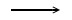# How many atoms does aluminum chloride contain?

Exercises for setting up reaction equations

When setting up reaction equations in chemistry (8th grade curriculum), you should follow these steps:

Reaction of an element of III. Main group with a halogen

Example:
Aluminum and chlorine react to form aluminum chloride

 1) Establish word equation.
 Aluminum + chlorineAluminum chloride

 2) Use symbols and formulas Important: Note that all gaseous elements (except noble gases) occur as diatomic elements !!
 Al + Cl2AlCl3

3) Establish reaction equation

Important: The number of atoms of an element must be the same on the left and right side of the reaction arrow.

 Note: Cl2 means that 2 atoms are bound in a molecule 2 AlCl3 means that 2 moles of AlCl3 are present. Note: In a chemical reaction, it is not the atoms that change; the atoms are simply linked to one another in a different way. (see Dalton's atomic theory)

It is said: 2 moles of aluminum react with 3 moles of chlorine to form 2 moles of aluminum chloridenext example